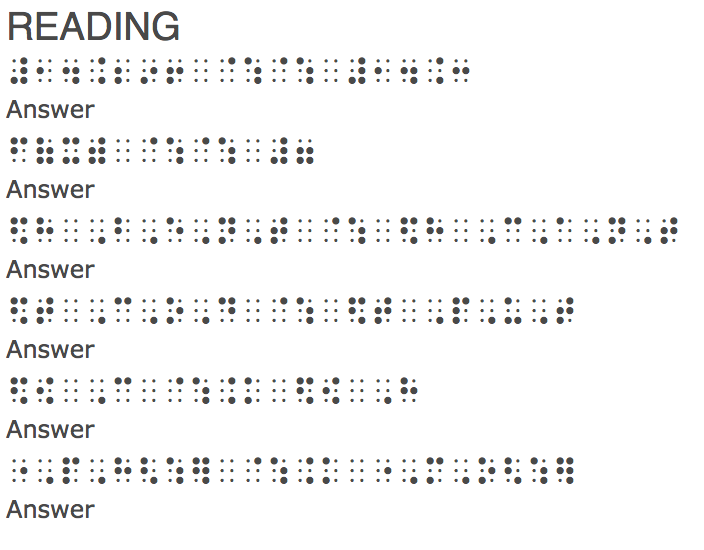Resource

# Nemeth Tutorial from APH

## Tutorial in Nemeth Code for braille notation of mathematics and science from APH (American Printing House for the Blind)

### What is Nemeth Code?

Nemeth Code is the standard code of braille notation used for math and science.  It was developed by Dr. Abraham Nemeth in 1946, and was adopted by BANA (Braille Authority of North America) in 1952 as the standard code to represent mathematic and scientific notation, using the 6-dot braille cell.

### How Can I Learn Nemeth Code?

The Nemeth Tutorial is a project with American Printing House for the Blind (APH) that is designed to teach students and teachers how to use the Nemeth code for all levels of mathematics, from introductory to advanced topics.

There are 11 chapters are organized by grade level, from 1st to 8th grade, and then by content area for algebra, geometry and advanced mathematics.

• 1.1 The Numeric Indicator
• 1.2 Punctuation
• 1.3 Comma
• 1.4 Plus and Minus Signs
• 1.5 Equals Sign
• 1.6 Signs of Comparison

• 2.1 Abbreviations
• 2.2 Decimal Point
• 2.3 Monetary Sign: Dollar and Cent

• 3.1 Multiplication Cross
• 3.2 Division
• 3.3 Parentheses
• 3.4 Simple Fractions
• 3.5 Omission Symbol
• 3.6 Roman Numerals
• 3.7 Mixed Fraction
• 3.8 Introduction to Nemeth Indicator

• 4.1 Variables
• 4.2 Shapes Omissions
• 4.3 Ratios and Percents
• 4.4 Degrees
• 4.5 Basic Geometric Symbols

• 5.1 Basic Exponents
• 5.2 Segments, Lines, Rays and their Relationship
• 5.3 Directed Signed
• 5.4 Ordered Pairs

• 6.1 Absolute Value
• 6.2 The Horizontal Bar – Repeating Decimals, Greater than or equal to, and Less than or equal to
• 6.3 Brackets and Braces

• 7.1 Complex Fractions

• 8.1 Real Numbers
• 8.2 Plus/Minus and Not Equal Sign

Chapter Nine: Algebra
• 9.1 Set Notations: Complements, Subsets, Union, Intersection
• 9.2 Ratios and Proportions
• 9.3 More Sets: Union, Intersections and the Infinity Symbol
• 9.4 Matrices
• 9.5 Factorial

Chapter Ten: Geometry
• 10.1 Signs of Comparison: Approximately Equal To, Similar To, Congruent To
• 10.2 Conjunction, Disjunction, Conditionals, and Biconditionals

• 11.1 Subscripts
• 11.2 Funtion Name Abbreviation
• 11.3 Vectors
• 11.4 Circles and Arcs